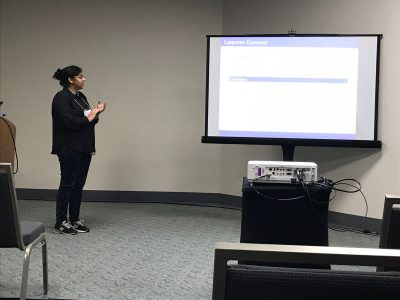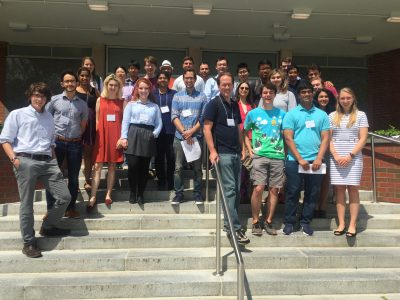# Fractional Gaussian fields on surfaces and graphs

Group Members: Tyler Campos, Andrew Gannon, Benjamin Hanzsek-Brill, Connor Marrs, Alexander Neuschotz, Trent Rabe and Ethan Winters.

Overview: We study and simulate on computers the fractional Gaussian fields and their discretizations on surfaces like the two-dimensional sphere or two-dimensional torus. The study of the maxima of those processes will be done and conjectures formulated concerning limit laws. Particular attention will be paid to log-correlated fields (the so-called Gaussian free field).

# Fair pricing and hedging under small perturbations of the numéraire on a finite probability space

### William Busching, Delphine Hintz, Oleksii Mostovyi, Alexey Pozdnyakov

• Fair Pricing and Hedging Under Small Perturbations of the Numéraire on a Finite Probability Space

Involve (2022), Vol. 15(4), pp. 649-668. [published version] [arXiv]

# Box-ball systems and RSK tableaux

Sém. Lothar. Combin.  85B  (2021), Art. 14, 12 pp.

Proceedings of the 33rd Conference on Formal Power
Series and Algebraic Combinatorics

Ben Drucker, Eli Garcia, and Rose Silver

A box-ball system is a collection of discrete time states representing a permutation,
on which there is an action called a BBS move. After a finite number of BBS moves
the system decomposes into a collection of soliton states; these are weakly
increasing and invariant under BBS moves. The students proved that when this
collection of soliton states is a Young tableau or coincides with a partition of a type
described by Robinson-Schensted (RS), then it is an RS insertion tableau. They also
studied the number of steps required to reach this state.

# Hedging by Sequential Regression in Generalized Discrete Models and the Follmer-Schweizer decomposition## Overview

In practice, financial models are not exact — as in any field, modeling based on real data introduces some degree of error. However, we must consider the effect error has on the calculations and assumptions we make on the model.  In complete markets, optimal hedging strategies can be found for derivative securities; for example, the recursive hedging formula introduced in Steven Shreve’s “Stochastic Calculus for Finance I” gives an exact expression in the binomial asset model, and as a result the unique arbitrage-free price can be computed at any time for any derivative security.

In incomplete markets this cannot be accomplished; one possibility for computing optimal hedging strategies is the method of sequential regression.  We considered this in discrete-time; in the (complete) binomial model we showed that the strategy of sequential regression introduced by Follmer and Schweizer  is equivalent to Shreve’s recursive hedging formula, and in the (incomplete) trinomial model we both explicitly computed the optimal hedging strategy predicted by the Follmer-Schweizer decomposition and we showed that the strategy is stable under small perturbations.

project page:

# Geodesic Interpolation on the Sierpinski Gasket

## Overview

Geodesics (shortest paths) on manifolds such as planes and spheres are well understood.  Geodesics on fractal sets such as the Sierpinski Triangle are much more complicated.  We begin by constructing algorithms for building shortest paths and provide explicit formulas for computing their lengths.  We then turn to the question of interpolation along geodesics—given two subsets of the Sierpinski Triangle, we “slide” points in one set along geodesics to the other set.  We construct a measure along the interpolated sets which formalizes a notion of the interpolation of a distribution of mass, and we prove interesting self-similarity relations about this measure.

# Math UConn REU at JMM 2018

Two of our REU (2017 Stochastics) participants, Raji Majumdar and Anthony Sisti, will be presenting posters Applications of Multiplicative LLN and CLT for Random Matrices and Black Scholes using the Central Limit Theorem on Friday, January 12 at the MAA Student Poster Session, and both of them will be giving talks on Saturday, January 13 at the AMS Contributed Paper Session on Research in Applied Mathematics by Undergraduate and Post-Baccalaureate Students.

Their travel to the 2018 JMM has been made possible with the support of the MAA and UConn’s OUR travel grants.# 5th Northeast Mathematics Undergraduate Research Mini-Symposium

### University of Connecticut, August 3rd, 2017

#### Participating Schools: Amherst, Smith, UConn and UMass

5th Mini-Symposium full program (2017)